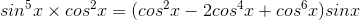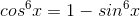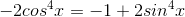# Quick simple indentity explanation needed

#### amd123

1. The problem statement, all variables and given/known data
When you have something likeand you wanna use the pythagorean's theorem identity but lets say i do it with the 3rd term in the parenthesis, then would this be validis this also valid?

2. Relevant equations

N/A

3. The attempt at a solution

N/A

#### praharmitra

no it would not be valid.

to verify this, cube and square sin(x)^2 + cos(x)^2 = 1, and check

#### yeongil

Heh, my students just learned proving identities, and they've been kicking them in the ***...
$$sin^{5}x cos^{2}x = (cos^{2}x - 2cos^{4}x + cos^{6}x)sin x$$
You do want to use the Pythagorean identity, but not in the first step. You can also start on either side, but I did from the left.

If you start from the left side, my hint would be to rewrite $$sin^{5}x cos^{2}x$$ using the properties of exponents so that it equals (everything else) times sin x.

01

### The Physics Forums Way

We Value Quality
• Topics based on mainstream science
• Proper English grammar and spelling
We Value Civility
• Positive and compassionate attitudes
• Patience while debating
We Value Productivity
• Disciplined to remain on-topic
• Recognition of own weaknesses
• Solo and co-op problem solving